# Roman numerals 2+

Add up the number writtens in Roman numerals. Write the results as a roman numbers.

Result

DCCLXXXVIII + DCCLXVII = (Correct answer is: MDLV)CCXXII + CMLXVIII = (Correct answer is: MCXC)DXXXIV + DCCCLXV = (Correct answer is: MCCCXCIX)CCCLXXXIV + CMXCVII = (Correct answer is: MCCCLXXXI)DCCXLVII + CCLXII = (Correct answer is: MIX)DVIII + CMXXVIII = (Correct answer is: MCDXXXVI)DCCCLXXX + DCCLXXXVII = (Correct answer is: MDCLXVII)CDXXVIII + DCXCII = (Correct answer is: MCXX)CCCLXXV + DCCXCI = (Correct answer is: MCLXVI)CDXXXVIII + CXXXVIII = (Correct answer is: DLXXVI)#### Solution:

DCCLXXXVIII + DCCLXVII = 788 + 767 = 1555 = MDLV
CCXXII + CMLXVIII = 222 + 968 = 1190 = MCXC
DXXXIV + DCCCLXV = 534 + 865 = 1399 = MCCCXCIX
CCCLXXXIV + CMXCVII = 384 + 997 = 1381 = MCCCLXXXI
DCCXLVII + CCLXII = 747 + 262 = 1009 = MIX
DVIII + CMXXVIII = 508 + 928 = 1436 = MCDXXXVI
DCCCLXXX + DCCLXXXVII = 880 + 787 = 1667 = MDCLXVII
CDXXVIII + DCXCII = 428 + 692 = 1120 = MCXX
CCCLXXV + DCCXCI = 375 + 791 = 1166 = MCLXVI
CDXXXVIII + CXXXVIII = 438 + 138 = 576 = DLXXVI

Leave us a comment of example and its solution (i.e. if it is still somewhat unclear...):Be the first to comment!## Next similar examples:

1. Roman numerals +Add up the number writtens in Roman numerals. Write the results as a decimal number.
2. Multiples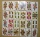What is the sum of the multiples of number 7 that are greater than 30 but less than 56?
3. ProductResult of the product of the numbers 1, 2, 3, 1, 2, 0 is:
4. SummandOne of the summands is 145. The second is 10 more. Determine the sum of the summands.Add this two mixed numbers: 1 5/6 + 2 2/11=
6. Operations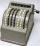Sum of the numbers 1.01 and 3.35 multiply by the difference of numbers 6.69 and 1.39.
7. Decimal expansion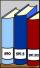Calculate: 2 . 1 + 0 . 10 + 7 . 10000 + 4 . 1000 + 6 . 100 + 0 . 100000 =
8. Tulips and daffodils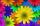Farm cultivated tulips and 211 units on 50 units more daffodils. How many spring flowers grown together?
9. CollectionMajka gave from her collection of calendars Hanke 15 calendars, Julke 6 calendars and Petke 10 calendars. Still remains 77 calendars. How many calendars had Majka in her collection at the beginning?
10. Car parking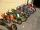The car park has 45 motorcycles and 25 cars. 14 cars went away and 2 arrived, then departs 12 motorcycles and 2 arrive. How many motorbikes and cars there are now?
11. School trip6.A class went on a trip. Train ticket cost 6 euros, cable car ticket 6 euro. How many euros must pay classroom teacher for 23 students?
12. Compare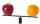Compare with characters >, <, =: 85.57 ? 80.83
13. This morningThis morning it was 80 degrees F outside the temperature increased 12 degrees F. What is the temperature now?
14. Roses and tulipsAt the florist are 50 tulips and 5 times less roses. How many flowers are in flower shop?Combine like terms 4c+c-7cProve whether you can construct a triangle ABC, if a=9 cm, b=10 cm, c=4 cm.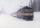The train passes part of the line for 95 minutes at speed 75 km/h. What speed would have to go in order to shorten the driving time of 20 minutes?